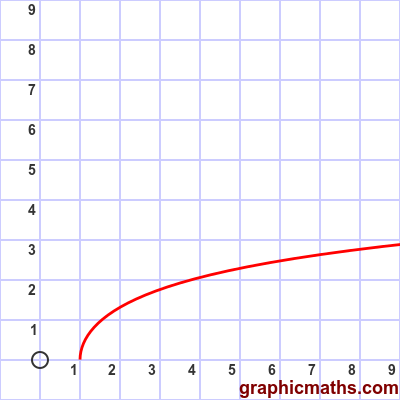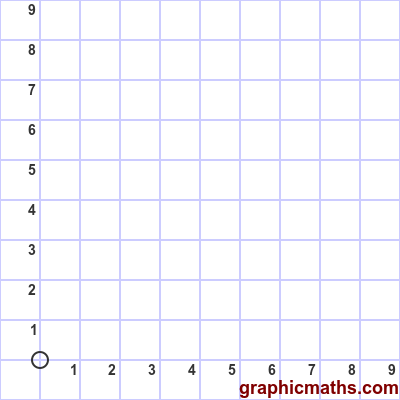# arcosh function

By Martin McBride, 2020-02-03
Tags: arcosh
Categories: hyperbolic functions

The arcosh function is a hyperbolic function. It is the inverse of the cosh, and is also known as the inverse hyperbolic cosine function.

Why is it called the arcosh rather than the arccosh? See here.

## Equation and graph

The arcosh function is defined as the inverse of cosh, ie if:

$$x = \cosh y$$

then:

$$y = \operatorname{arcosh} x$$

There is a also a formula for finding arcosh directly:

$$\operatorname{arcosh} x= \ln{x+{\sqrt {x^{2}-1}}}$$

Here is a graph of the function:The function is valid for x >= 1.

## arcosh as inverse of cosh

This animation illustrates the relationship between the cosh function and the arcosh function:The first, blue, curve is the cosh function.

The grey dashed line is the line $y=x$.

The second, red, curve is the arcosh function. As with any inverse function, it is identical to the original function reflected in the line $y=x$.

## Logarithm formula for arcosh

arcosh can be calculated directly, using a logarithm function, like this:

$$\operatorname{arcosh}{x}= \ln({x+{\sqrt {x^{2}-1}}})$$

Here is a proof of the logarithm formula for arcosh. It is very similar to the proof for arsinh.

We will use:

$$u = \operatorname{arcosh}{x}$$

The cosh of u will be x, because cosh is the inverse of arcosh:

$$x = \cosh {u}$$

One form of the formula for cosh is:

$$\cosh{u} = \frac{e^{2u}+1}{2e^{u}}$$

This gives us:

$$x = \cosh {u} = \frac{e^{2u}+1}{2e^{u}}$$

Multiplying both sides by $2e^{u}$ gives:

$$2 x e^{u} = e^{2u} + 1$$

This is a quadratic in $e^{u}$ (using the fact that $e^{2u}=(e^{u})^2$):

$$0 = (e^{u})^2 -2 x (e^{u})+1$$

We use the quadratic formula with $a=1$, $b=-2x$, $c=1$:

$$e^{u} = \frac{-b\pm\sqrt{b^2-4ac}}{2a} = \frac{2x\pm\sqrt{4{x^2}-4}}{2}$$

Simplifying and taking the positive solution (since $e^{u}$ must be positive) gives:

$$e^{u} = x+\sqrt{x^2-1}$$

Taking the logarithm of both sides gives:

$$ln(e^{u}) = u = ln(x+\sqrt{x^2-1})$$

And since u is $\operatorname{arcosh}{x}$ this gives:

$$\operatorname{arcosh}{x}= \ln({x+{\sqrt {x^{2}-1}}})$$